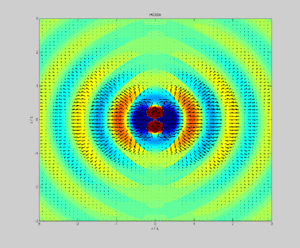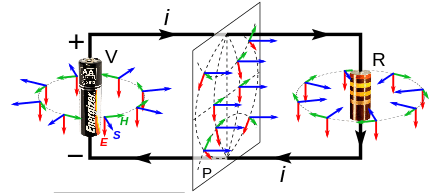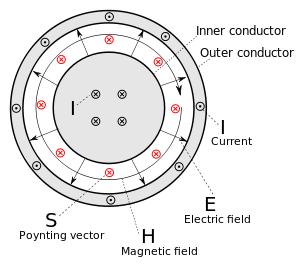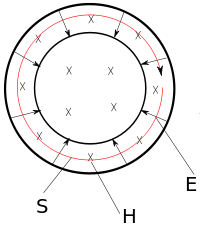# Poynting vectorDipole radiation of a dipole vertically in the page showing electric field strength (colour) and Poynting vector (arrows) in the plane of the page.

In physics, the Poynting vector represents the directional energy flux density (the rate of energy transfer per unit area) of an electromagnetic field. The SI unit of the Poynting vector is the watt per square metre (W/m2). It is named after its discoverer John Henry Poynting who first derived it in 1884.:132 Oliver Heaviside:132 and Nikolay Umov:147 also independently discovered the Poynting vector.

## Definition

In Poynting's original paper and in many textbooks, the Poynting vector is defined aswhere bold letters represent vectors and

This expression is often called the Abraham form. The Poynting vector is usually denoted by S or N.

In the "microscopic" version of Maxwell's equations, this definition must be replaced by a definition in terms of the electric field E and the magnetic flux density B (it is described later in the article).

It is also possible to combine the electric displacement field D with the magnetic flux density B to get the Minkowski form of the Poynting vector, or use D and H to construct yet another version. The choice has been controversial: Pfeifer et al. summarize and to a certain extent resolve the century-long dispute between proponents of the Abraham and Minkowski forms (see Abraham–Minkowski controversy).

The Poynting vector represents the particular case of an energy flux vector for electromagnetic energy. However, any type of energy has its direction of movement in space, as well as its density, so energy flux vectors can be defined for other types of energy as well, e.g., for mechanical energy. The Umov–Poynting vector discovered by Nikolay Umov in 1874 describes energy flux in liquid and elastic media in a completely generalized view.

## InterpretationA DC circuit consisting of a battery (V) and resistor (R), showing the direction of the Poynting vector (S, blue) in the space surrounding it, along with the fields it is derived from; the electric field (E, red) and the magnetic field (H, green). In the region around the battery the Poynting vector is directed outward, indicating power flowing out of the battery into the fields; in the region around the resistor the vector is directed inward, indicating field power flowing into the resistor. Across any plane P between the battery and resistor, the Poynting flux is in the direction of the resistor.

The Poynting vector appears in Poynting's theorem (see that article for the derivation), an energy-conservation law:where Jf is the current density of free charges and u is the electromagnetic energy density for linear, nondispersive materials, given bywhere

• E is the electric field;
• D is the electric displacement field;
• B is the magnetic flux density;
• H is the magnetic field.:258–260

The first term in the right-hand side represents the electromagnetic energy flow into a small volume, while the second term subtracts the work done by the field on free electrical currents, which thereby exits from electromagnetic energy as dissipation, heat, etc. In this definition, bound electrical currents are not included in this term, and instead contribute to S and u.

For linear, nondispersive and isotropic (for simplicity) materials, the constitutive relations can be written aswhere

Here ε and μ are scalar, real-valued constants independent of position, direction, and frequency.

In principle, this limits Poynting's theorem in this form to fields in vacuum and nondispersive linear materials. A generalization to dispersive materials is possible under certain circumstances at the cost of additional terms.:262–264

## Formulation in terms of microscopic fields

The "microscopic" version of Maxwell's equations admits only the fundamental fields E and B, without a built-in model of material media. Only the vacuum permittivity and permeability are used, and there is no D or H. When this model is used, the Poynting vector is defined aswhere

The corresponding form of Poynting's theorem iswhere J is the total current density and the energy density u is given bywhere ε0 is the vacuum permittivity. It can be derived directly from Maxwell's equations in terms of total charge and current and the Lorentz force law only.

The two alternative definitions of the Poynting vector are equal in vacuum or in non-magnetic materials, where B = μ0H. In all other cases, they differ in that S = (1/μ0) E × B and the corresponding u are purely radiative, since the dissipation term JE covers the total current, while the E × H definition has contributions from bound currents which are then excluded from the dissipation term.

Since only the microscopic fields E and B occur in the derivation of S = (1/μ0) E × B, assumptions about any material present are completely avoided, and Poynting vector and theorem are universally valid, in vacuum as in all kinds of material. This is especially true for the electromagnetic energy density, in contrast to the "macroscopic" form E × H.

## Time-averaged Poynting vector

The above form for the Poynting vector represents the instantaneous power flow due to instantaneous electric and magnetic fields. More commonly, problems in electromagnetics are solved in terms of sinusoidally varying fields at a specified frequency. The results can then be applied more generally, for instance, by representing incoherent radiation as a superposition of such waves at different frequencies and with fluctuating amplitudes.

We would thus not be considering the instantaneous E(t) and H(t) used above, but rather a complex (vector) amplitude for each which describes a coherent wave's phase (as well as amplitude) using phasor notation. Note that these complex amplitude vectors are not functions of time, as they are understood to refer to oscillations over all time. A phasor such asis understood to signify a sinusoidally varying field whose instantaneous amplitude E(t) follows the real part ofwhere ω is the (radian) frequency of the sinusoidal wave being considered.

In the time domain it will be seen that the instantaneous power flow will be fluctuating at a frequency of 2ω. But what is normally of interest is the average power flow in which those fluctuations are not considered. In the math below, this is accomplished by integrating over a full cycle. The following quantity, still referred to as a "Poynting vector", is expressed directly in terms of the phasors as:where * denotes the complex conjugate. The time-averaged power flow (according to the instantaneous Poynting vector averaged over a full cycle, for instance) is then given by the real part of. The imaginary part is usually ignored, however it signifies "reactive power" such as the interference due to a standing wave or the near field of an antenna. In a single electromagnetic plane wave (rather than a standing wave which can be described as two such waves travelling in opposite directions), E and H are exactly in phase, sois simply a real number according to the above definition.

The equivalence ofto the time-average of the instantaneous Poynting vector S can be shown as follows.The average of the instantaneous Poynting vector S over time is given by:The second term is the double-frequency component having an average value of zero, so we find:According to some conventions the factor of 1/2 in the above definition may be left out. Multiplication by 1/2 is required to properly describe the power flow since the magnitudes ofandrefer to the peak fields of the oscillating quantities. If rather the fields are described in terms of their root mean square (rms) values (which are each smaller by the factor), then the correct average power flow is obtained without multiplication by 1/2.

## Examples and applications

### Coaxial cablePoynting vector in a coaxial cable, shown in red.

For example, the Poynting vector within the dielectric insulator of a coaxial cable is nearly parallel to the wire axis (assuming no fields outside the cable and a wavelength longer than the diameter of the cable, including DC). Electrical energy delivered to the load is flowing entirely through the dielectric between the conductors. Very little energy flows in the conductors themselves, since the electric field strength is nearly zero. The energy flowing in the conductors flows radially into the conductors and accounts for energy lost to resistive heating of the conductor. No energy flows outside the cable, either, since there the magnetic fields of inner and outer conductors cancel to zero.

### Resistive dissipation

If a conductor has significant resistance, then, near the surface of that conductor, the Poynting vector would be tilted toward and impinge upon the conductor. Once the Poynting vector enters the conductor, it is bent to a direction that is almost perpendicular to the surface.:61 This is a consequence of Snell's law and the very slow speed of light inside a conductor. The definition and computation of the speed of light in a conductor can be given.:402 Inside the conductor, the Poynting vector represents energy flow from the electromagnetic field into the wire, producing resistive Joule heating in the wire. For a derivation that starts with Snell's law see Reitz page 454.:454

### Plane waves

In a propagating sinusoidal linearly polarized electromagnetic plane wave of a fixed frequency, the Poynting vector always points in the direction of propagation while oscillating in magnitude. The time-averaged magnitude of the Poynting vector is found as above to be:where Em is the complex amplitude of the electric field and η is the characteristic impedance of the transmission medium, or just η0 = 377Ω for a plane wave in free space. This directly follows from the above expression for the average Poynting vector using phasor quantities, and the fact that in a plane wave the magnetic fieldis equal to the electric fielddivided by η (and thus exactly in phase).

In optics, the time-averaged value of the radiated flux is technically known as the irradiance, more often simply referred to as the intensity.

The density of the linear momentum of the electromagnetic field is S/c2 where S is the magnitude of the Poynting vector and c is the speed of light in free space. The radiation pressure exerted by an electromagnetic wave on the surface of a target is given by### Static fieldsPoynting vector in a static field, where E is the electric field, H the magnetic field, and S the Poynting vector.

The consideration of the Poynting vector in static fields shows the relativistic nature of the Maxwell equations and allows a better understanding of the magnetic component of the Lorentz force, q(v × B). To illustrate, the accompanying picture is considered, which describes the Poynting vector in a cylindrical capacitor, which is located in an H field (pointing into the page) generated by a permanent magnet. Although there are only static electric and magnetic fields, the calculation of the Poynting vector produces a clockwise circular flow of electromagnetic energy, with no beginning or end.

While the circulating energy flow may seem nonsensical or paradoxical, it is necessary to maintain conservation of momentum. Momentum density is proportional to energy flow density, so the circulating flow of energy contains an angular momentum. This is the cause of the magnetic component of the Lorentz force which occurs when the capacitor is discharged. During discharge, the angular momentum contained in the energy flow is depleted as it is transferred to the charges of the discharge current crossing the magnetic field.

## Adding the curl of a vector field

The Poynting vector occurs in Poynting's theorem only through its divergence ∇ ⋅ S, that is, it is only required that the surface integral of the Poynting vector around a closed surface describe the net flow of electromagnetic energy into or out of the enclosed volume. This means that adding a field to S which has zero divergence will result in a field which satisfies this required property of a Poynting vector field according to Poynting's theorem. Since the divergence of any curl is zero, one can add the curl of any vector field to the Poynting vector and the resulting vector field S' will still satisfy Poynting's theorem.:258–260

However the theory of special relativity, in which energy and momentum are defined locally and invariantly via the stress–energy tensor, shows that the above given expression for the Poynting vector is unique.:258–260,605–612

It is generally argued that Maxwell equations are manifestly Lorentz covariant while the electromagnetic (EM) stress-energy tensor follows from the Maxwell equations; thus the EM momentum defined from the EM tensor certainly respects the principle of relativity. However a recent article indicates that "such an argument is based on an incomplete understanding of the relativity principle", and states that the EM stress-energy tensor is not sufficient to define EM momentum correctly. The study also claims that Poynting vector does not represent the EM power flow (energy flux density vector) for a plane wave in an anisotropic medium if the Poynting vector is not parallel to the wave vector, and states "this conclusion is clearly supported by Fermat's principle and special theory of relativity".

1. Stratton, Julius Adams (1941). Electromagnetic Theory (1st ed.). New York: McGraw-Hill. ISBN 978-0-470-13153-4.
2. Turowski, Janusz; Turowski, Marek (2014). Engineering Electrodynamics: Electric Machine, Transformer, and Power Equipment Design (1st ed.). Boca Raton, Florida: CRC Press. ISBN 978-1-4665-8932-2.
3. Poynting, John Henry (1884). "On the Transfer of Energy in the Electromagnetic Field". Philosophical Transactions of the Royal Society of London. 175: 343–361. doi:10.1098/rstl.1884.0016.
4. Grant, Ian S.; Phillips, William R. (1990). Electromagnetism (2nd ed.). New York: John Wiley & Sons. ISBN 978-0-471-92712-9.
5. Griffiths, David J. (2012). Introduction to Electrodynamics (3rd ed.). Boston: Addison-Wesley. ISBN 978-0-321-85656-2.
6. Kinsler, Paul; Favaro, Alberto; McCall, Martin W. (2009). "Four Poynting Theorems". European Journal of Physics. 30 (5): 983. arXiv:0908.1721. Bibcode:2009EJPh...30..983K. doi:10.1088/0143-0807/30/5/007.
7. Pfeifer, Robert N. C.; Nieminen, Timo A.; Heckenberg, Norman R.; Rubinsztein-Dunlop, Halina (2007). "Momentum of an Electromagnetic Wave in Dielectric Media". Reviews of Modern Physics. 79 (4): 1197. arXiv:0710.0461. Bibcode:2007RvMP...79.1197P. doi:10.1103/RevModPhys.79.1197.
8. Umov, Nikolay Alekseevich (1874). "Ein Theorem über die Wechselwirkungen in Endlichen Entfernungen". Zeitschrift für Mathematik und Physik. 19: 97–114.
9. Jackson, John David (1998). Classical Electrodynamics (3rd ed.). New York: John Wiley & Sons. ISBN 978-0-471-30932-1.
10. Richter, Felix; Florian, Matthias; Henneberger, Klaus (2008). "Poynting's Theorem and Energy Conservation in the Propagation of Light in Bounded Media". Europhysics Letters Association. 81 (6): 67005. arXiv:0710.0515. Bibcode:2008EL.....8167005R. doi:10.1209/0295-5075/81/67005.
11. Harrington, Roger F. (2001). Time-Harmonic Electromagnetic Fields (2nd ed.). McGraw-Hill. ISBN 978-0-471-20806-8.
12. Hayt, William (2011). Engineering Electromagnetics (4th ed.). New York: McGraw-Hill. ISBN 978-0-07-338066-7.
13. Reitz, John R.; Milford, Frederick J.; Christy, Robert W. (2008). Foundations of Electromagnetic Theory (4th ed.). Boston: Addison-Wesley. ISBN 978-0-321-58174-7.
14. Feynman, Richard Phillips (2011). The Feynman Lectures on Physics. Vol. II: Mainly Electromagnetism and Matter (The New Millennium ed.). New York: Basic Books. ISBN 978-0-465-02494-0.
15. Wang, C. (2015). "Self-consistent theory for a plane wave in a moving medium and light-momentum criterion". Canadian Journal of Physics. 93 (12): 1510. arXiv:1409.5807. Bibcode:2015CaJPh..93.1510W. doi:10.1139/cjp-2015-0167.
16. Wang, C. (2015). "Electromagnetic Power Flow, Fermat's Principle, and Special Theory of Relativity". Optik. 126 (20): 2703. doi:10.1016/j.ijleo.2015.06.053.